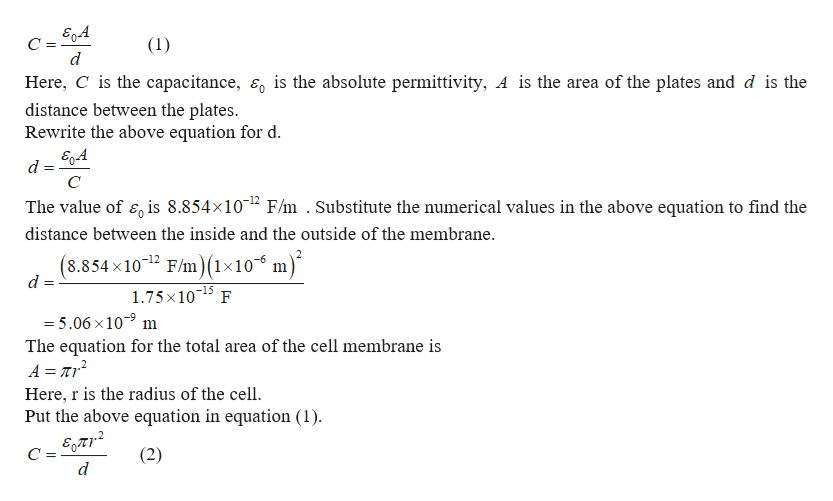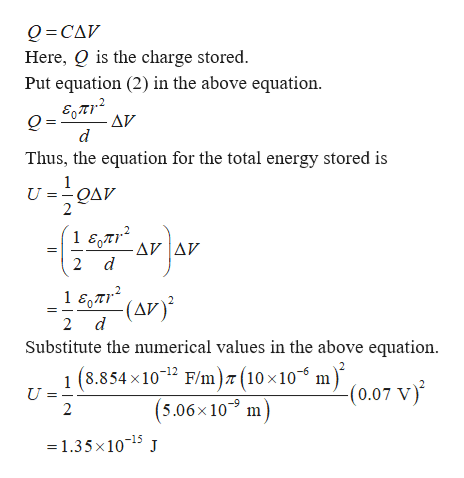# A capacitor stores a separation of charge. To separate the charges on a capacitor, you have to move charges against where the E field wants to push them (the oppositely charges on the two plates are attracted and want to move together), so charging takes work. That work becomes stored energy -- just like carrying water up a hill so that you can let it roll down at a later time and turn a generator. The total amount of energy stored in a capacitor is ½|Q||ΔV|. We'll use this to determine the amount of energy stored in a cell membrane.In discussion, we found that a cell membrane maintains a potential difference of about 70 mV (0.07 V) between the inside and outside of the membrane. We also found that a 1 μm by 1 μm section of the membrane has a capacitance of about 1.75  10-15 F.The radius of the cell is 10 μm. 4. What is the total energy stored in the full cell membrane?The answer is NOT 1.539e-13, 8.56e-18, or 4.28e-18.

Question

A capacitor stores a separation of charge. To separate the charges on a capacitor, you have to move charges against where the E field wants to push them (the oppositely charges on the two plates are attracted and want to move together), so charging takes work. That work becomes stored energy -- just like carrying water up a hill so that you can let it roll down at a later time and turn a generator. The total amount of energy stored in a capacitor is ½|Q||ΔV|. We'll use this to determine the amount of energy stored in a cell membrane.

In discussion, we found that a cell membrane maintains a potential difference of about 70 mV (0.07 V) between the inside and outside of the membrane. We also found that a 1 μm by 1 μm section of the membrane has a capacitance of about 1.75  10-15 F.

The radius of the cell is 10 μm.

4. What is the total energy stored in the full cell membrane?

The answer is NOT 1.539e-13, 8.56e-18, or 4.28e-18.

check_circleExpert Solution
Step 1

The equation for the capacitance is given byhelp_outlineImage TranscriptioncloseE0.4 C = (1) d Here, C is the capacitance, E is the absolute permittivity, A is the area of the plates and d is the distance between the plates. Rewrite the above equation for d. d = C The value of 5 is 8.854x102 F/m . Substitute the numerical values in the above equation to find the distance between the inside and the outside of the membrane. (8.854 x1012 F/m)(1x10 d m) 1.75x1015 F = 5.06 x 10 m The equation for the total area of the cell membrane is A = r2 Here, r is the radius of the cell Put the above equation in equation (1) C = d (2) fullscreen
Step 2

The equation for the charge store...help_outlineImage TranscriptioncloseQ CAV Here, Q is the charge stored. Put equation (2) in the above equation. AV d Thus, the equation for the total energy stored is UOAV - AV Δν 2 d 1 57(AV 2 d Substitute the numerical values in the above equation 8.854 x1012 F/m) (10 x10" m) (0.07 V) 1 ( U 2 (5.06x10 m =1.35x1015 J fullscreen

### Want to see the full answer?

See Solution

#### Want to see this answer and more?

Solutions are written by subject experts who are available 24/7. Questions are typically answered within 1 hour*

See Solution
*Response times may vary by subject and question
Tagged in

### Physics